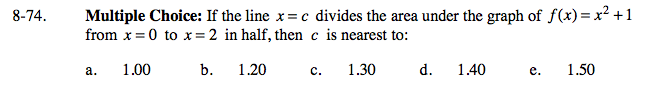### Home > CALC > Chapter 8 > Lesson 8.2.1 > Problem8-74

8-74.
1. Multiple Choice: If the line x = c divides the area under the graph of f(x) = x2 + 1 from x = 0 to x = 2 in half, then c is nearest to: Homework Help ✎

1. 1.00

2. 1.20

3. 1.30

4. 1.40

5. 1.50